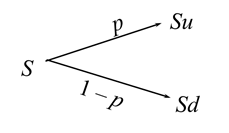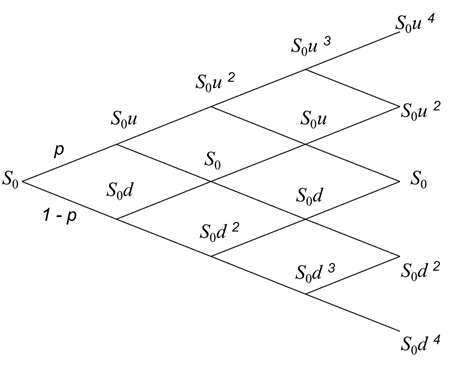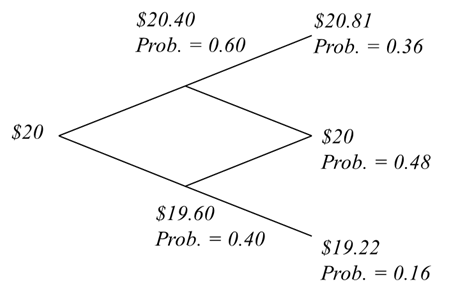# Stock Price Movement Using a Binomial Tree

The future price movement of a stock can be approximated using a binomial tree.

Let’s say the current stock price is S. The price of the stock can either move up or move down. We will refer to the up movement as u and the down movement as d. A down movement d will then be given by d where d is assumed to be equal to 1/u. We also need the probability of an up move (p) and the probability of a down move (1 - p).

The following diagram shows the price movement in a small time period Dt.From each node at Su and Sd, the prices can again take two possible paths.

• Su can move to Suu or Sud. Note that Sud will be the same as S (S*u*1/u).
• Sd can move to Sdu (or just S) or Sdd.

This way a binomial tree can be built for multiple periods. The following diagram shows the binomial tree for 4 periods.Let’s take an example to understand these values.

Initial stock price, S = $20 u = 1.02 d = 1/1.02 Probability of up move, p = 0.60 Probability of down move, (1-p) = 0.40 The possible stock values for period 1: • Su = 20*1.02 = 20.40 with a probability of 0.60 • Sd = 19.60 with a probability of 0.40 The possible stock values for period 2: • Suu = 20.81 with a probability of 0.60*0.60 = 0.36 • S =$20 with a probability of 2*0.60*0.40 = 0.48
• Sdd = 19.22 with a probability 0.40*0.40 = 0.16

Similarly we can calculate the values for period 3 and period 4. Binomial trees have their application in pricing options and other financial concepts.

The following diagram shows this binomial tree upto two periods.